# Selina Solutions Concise Maths Class 7 Chapter 11: Fundamental Concepts (Including Fundamental Operations) Exercise 11B

Selina Solutions Concise Maths Class 7 Chapter 11 Fundamental Concepts (Including Fundamental Operations) Exercise 11B has answers prepared by a set of expert teachers at BYJU’S. The addition and subtraction of like and unlike terms are the important concepts discussed under this exercise. The solutions contain explanations in an interactive manner to improve interest among students. For further hold on the concepts, students can download Selina Solutions Concise Maths Class 7 Chapter 11 Fundamental Concepts (Including Fundamental Operations) Exercise 11B PDF, from the links which are available below.

## Selina Solutions Concise Maths Class 7 Chapter 11: Fundamental Concepts (Including Fundamental Operations) Exercise 11B Download PDF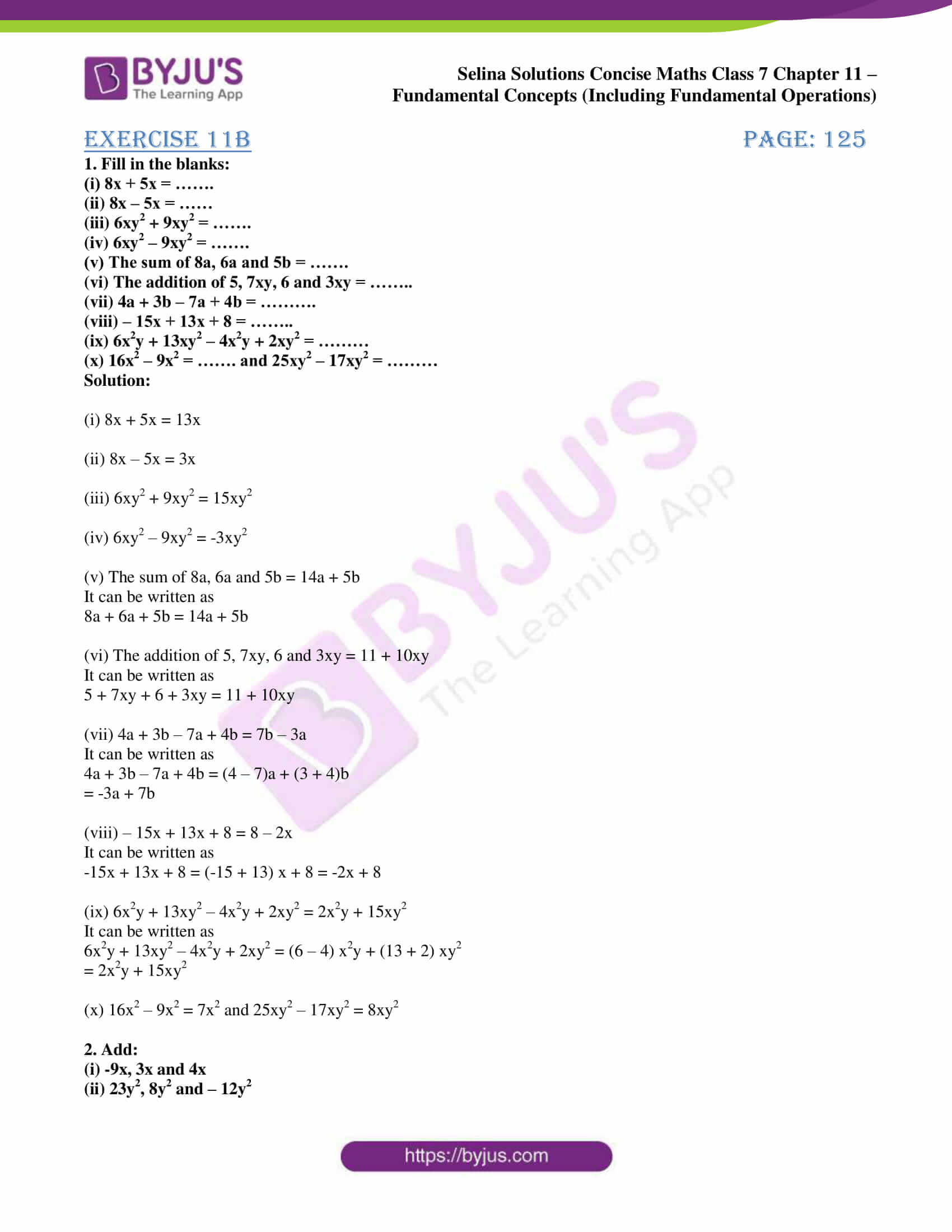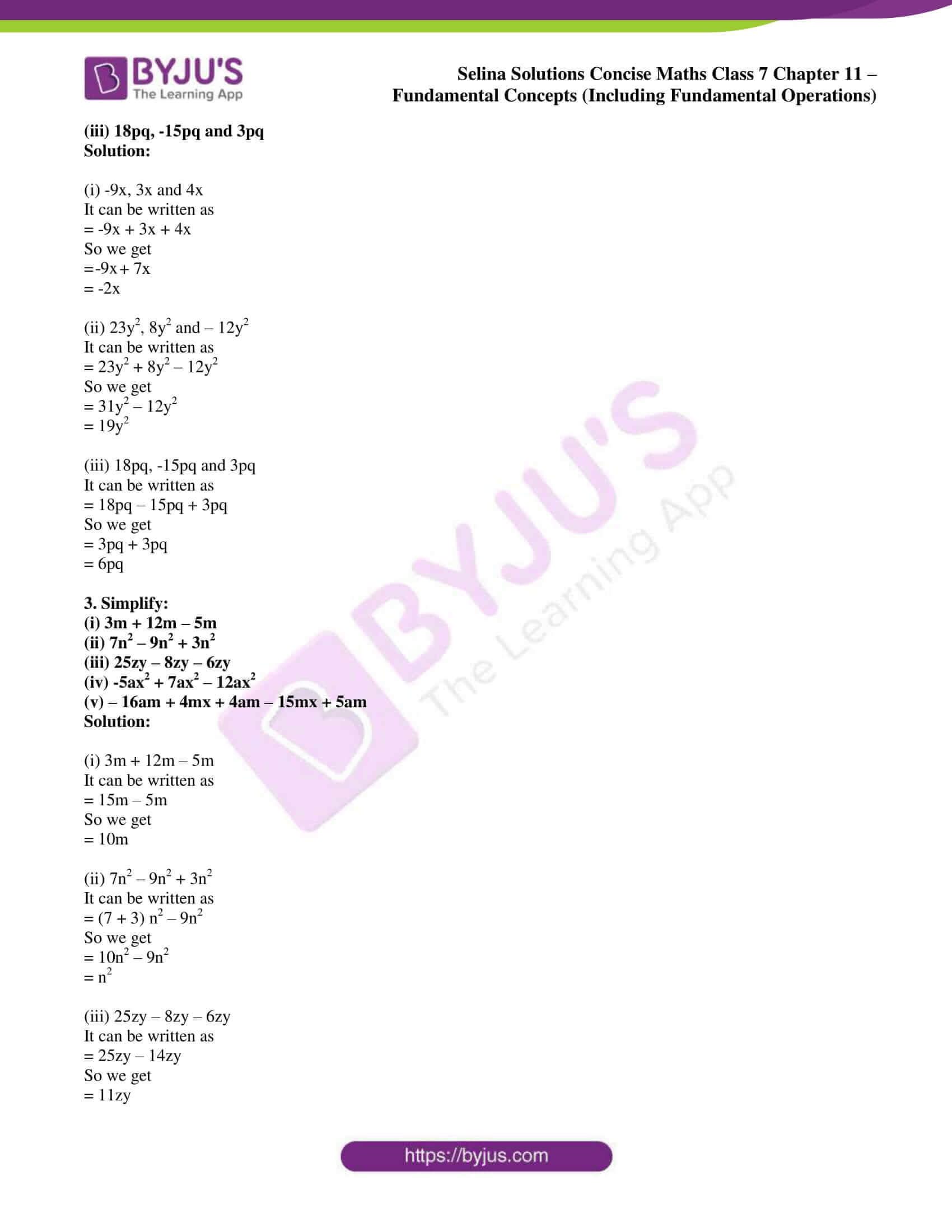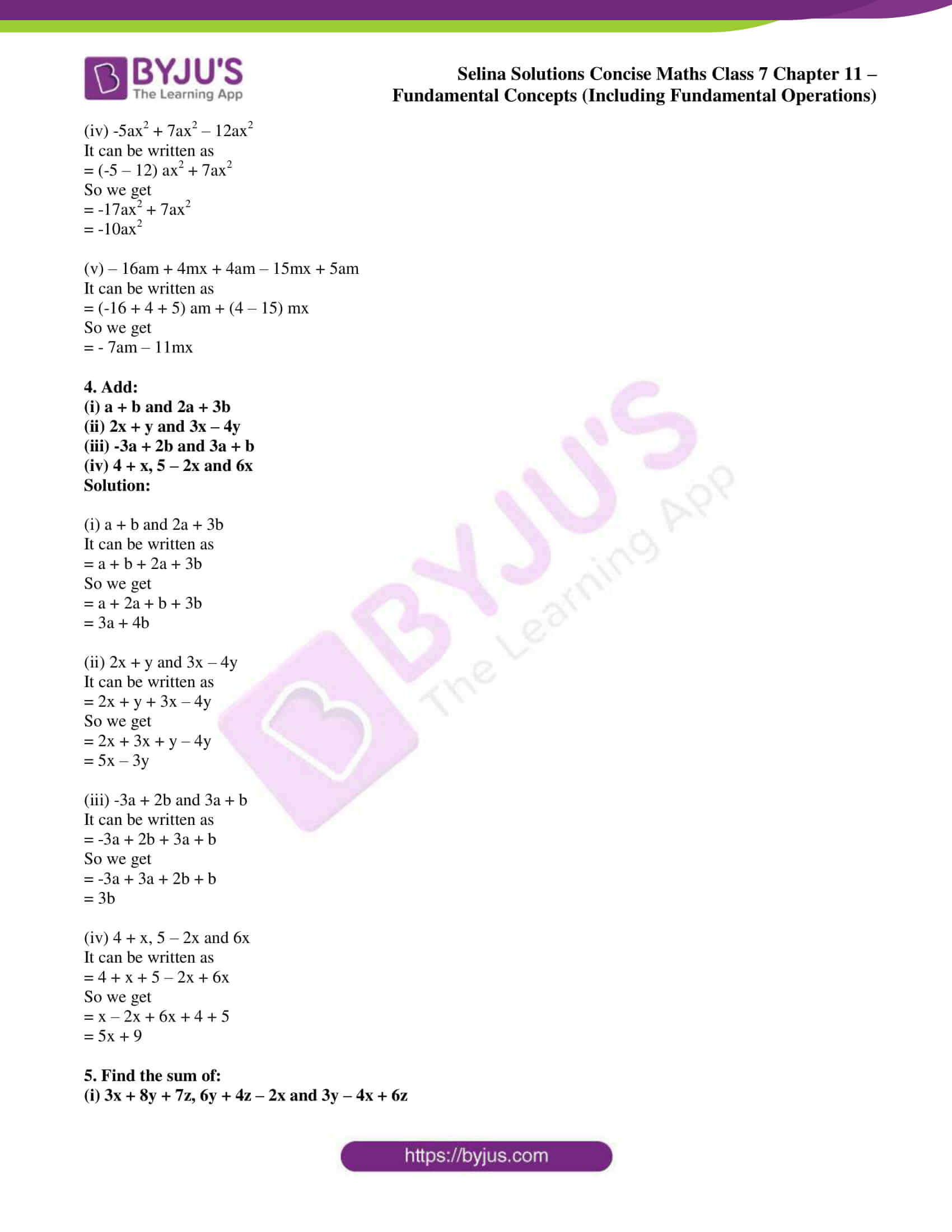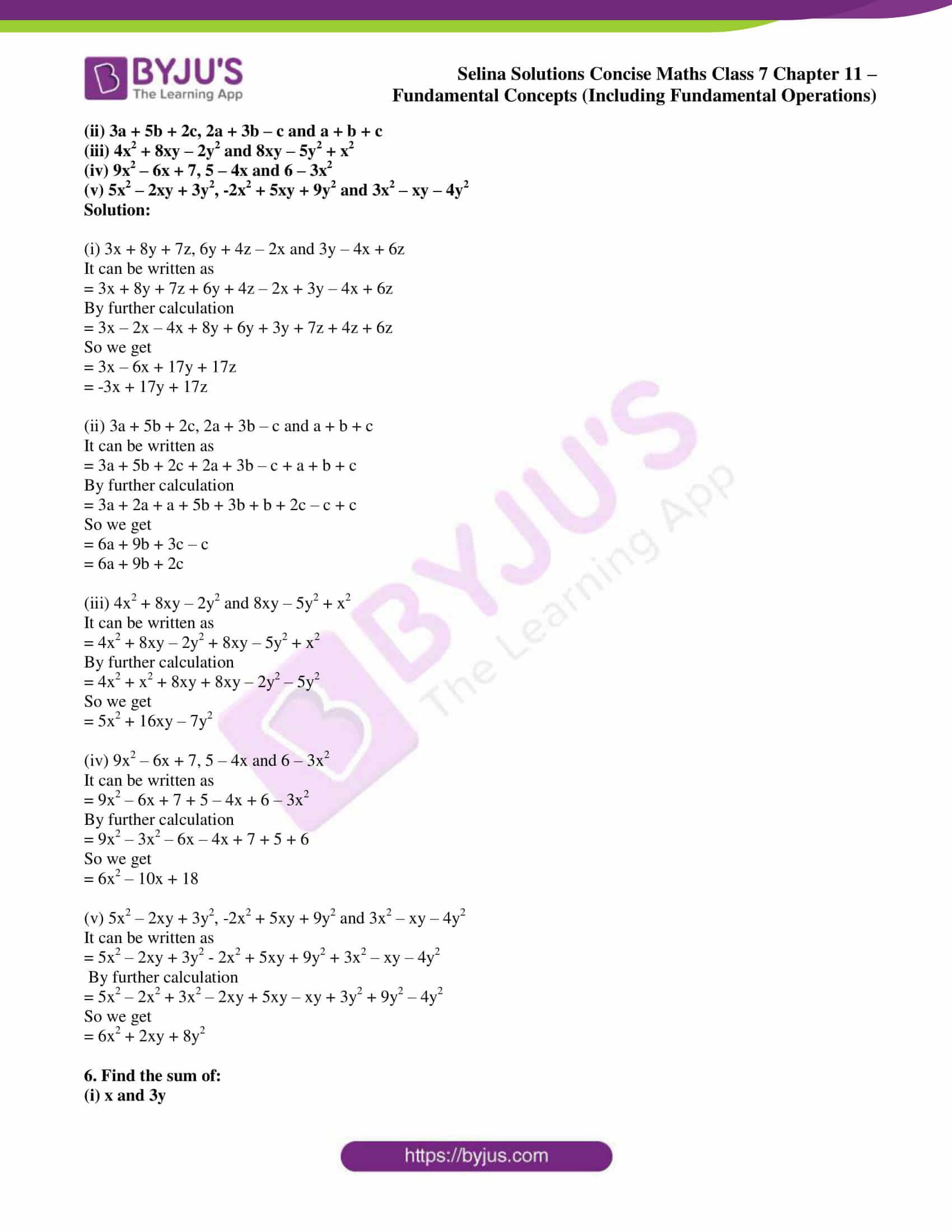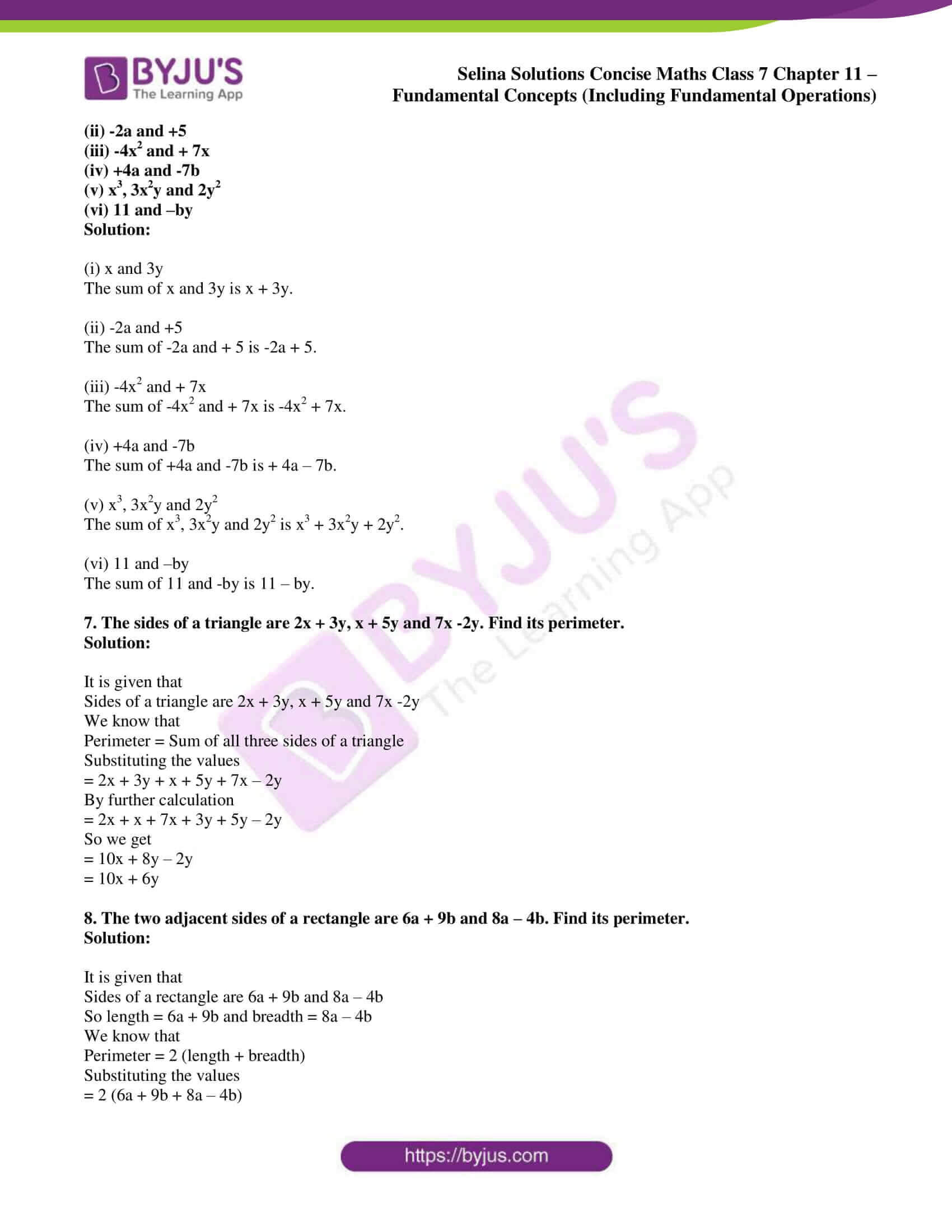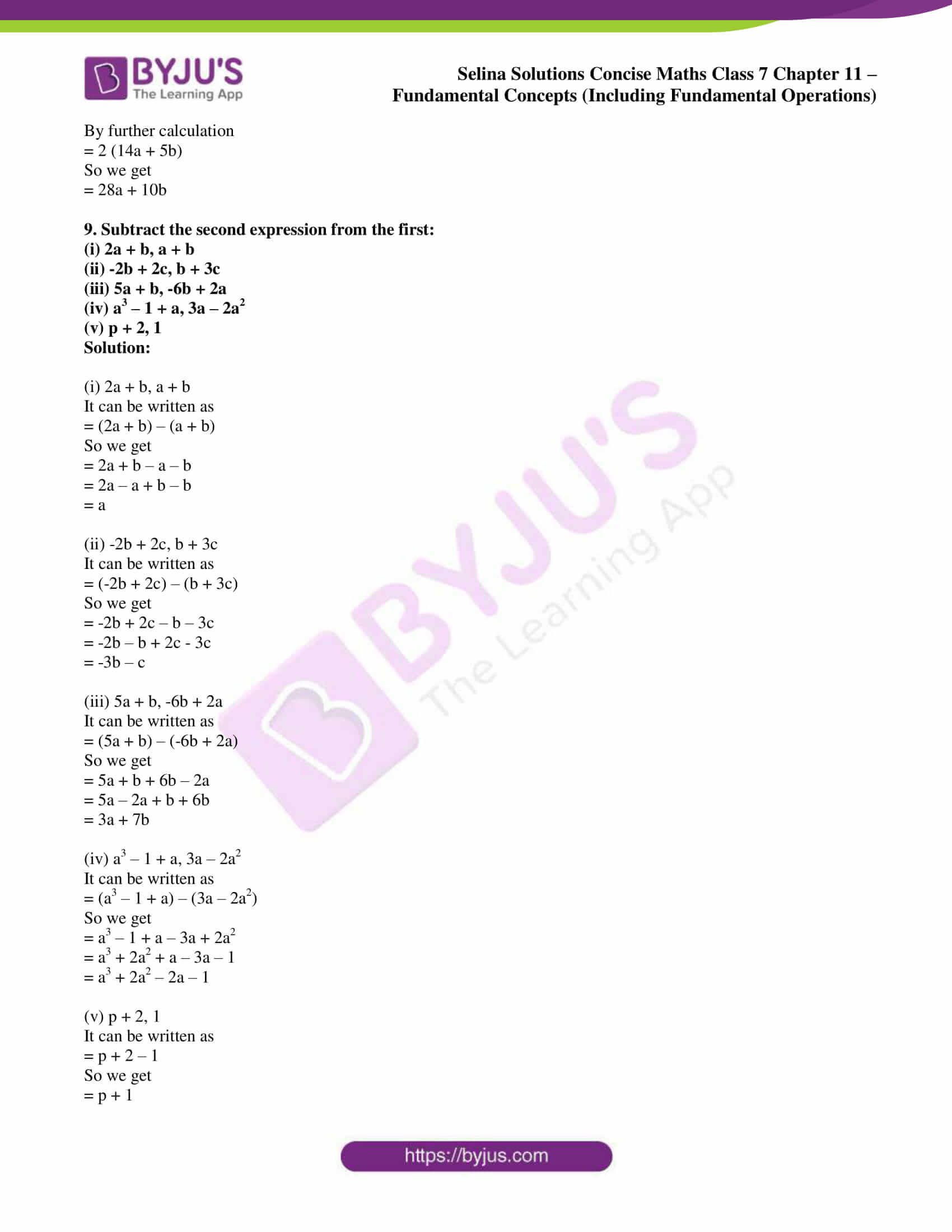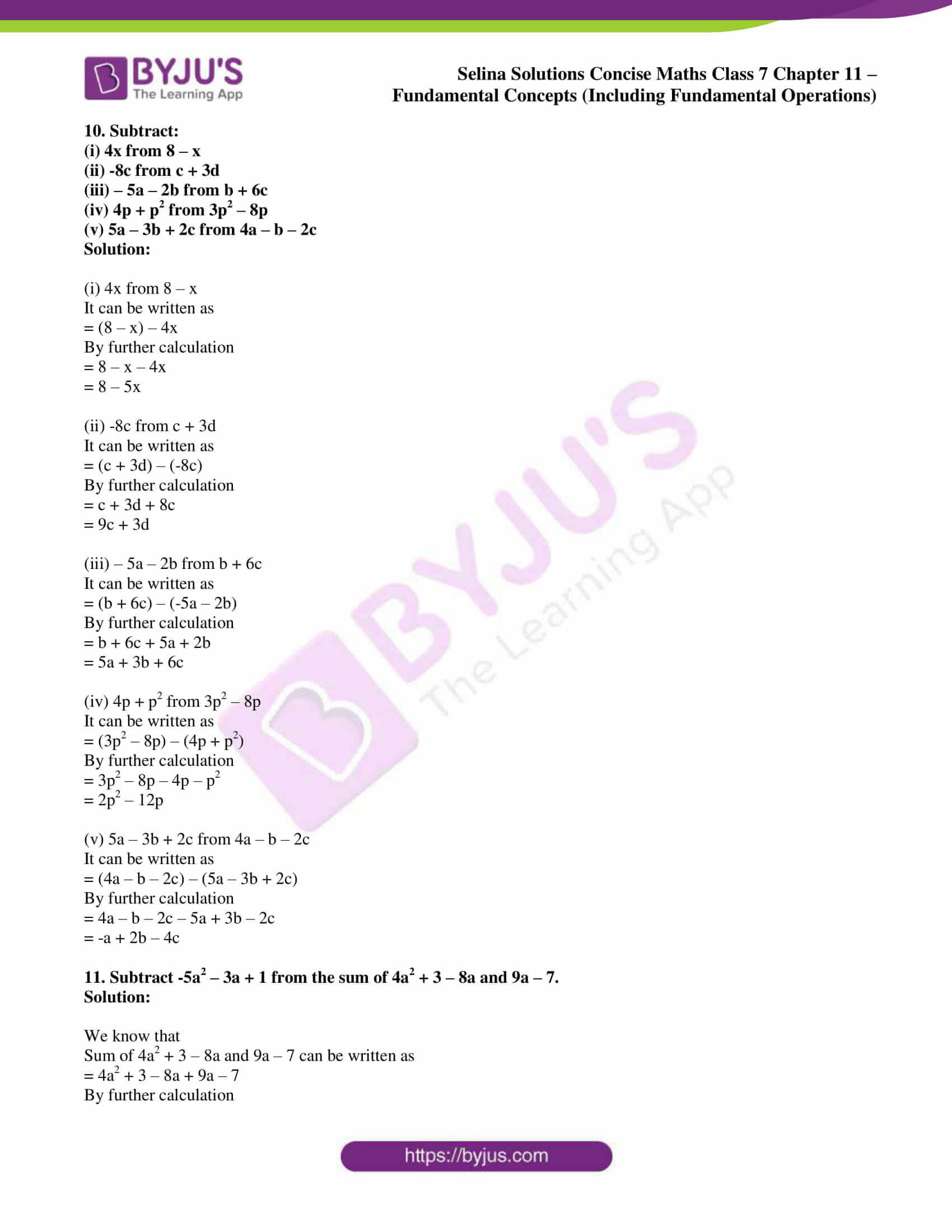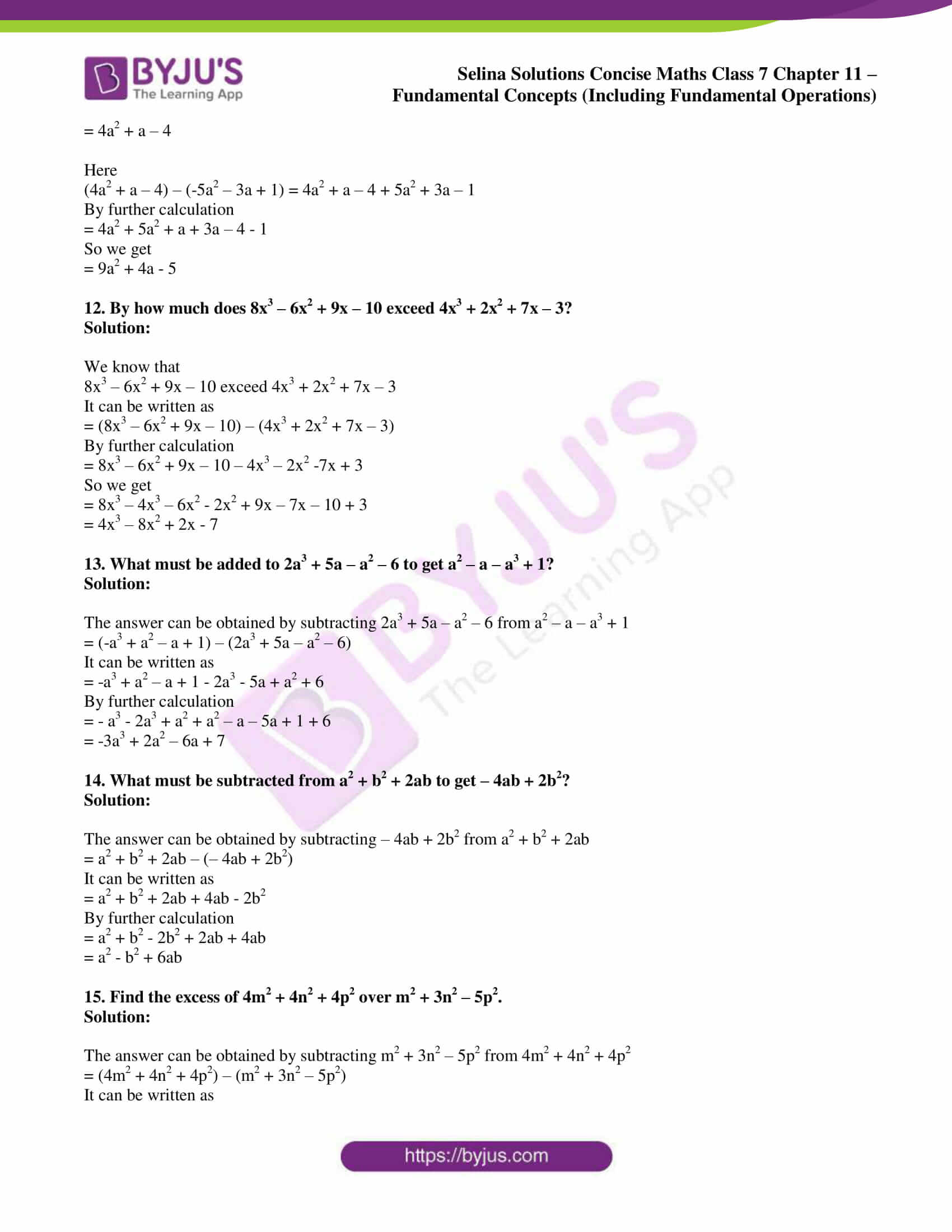### Access other exercises of Selina Solutions Concise Maths Class 7 Chapter 11: Fundamental Concepts (Including Fundamental Operations)

Exercise 11A Solutions

Exercise 11C Solutions

Exercise 11D Solutions

Exercise 11E Solutions

Exercise 11F Solutions

### Access Selina Solutions Concise Maths Class 7 Chapter 11: Fundamental Concepts (Including Fundamental Operations) Exercise 11B

#### Exercise 11B page: 125

1. Fill in the blanks:

(i) 8x + 5x = …….

(ii) 8x – 5x = ……

(iii) 6xy2 + 9xy2 = …….

(iv) 6xy2 – 9xy2 = …….

(v) The sum of 8a, 6a and 5b = …….

(vi) The addition of 5, 7xy, 6 and 3xy = ……..

(vii) 4a + 3b – 7a + 4b = ……….

(viii) – 15x + 13x + 8 = ……..

(ix) 6x2y + 13xy2 – 4x2y + 2xy2 = ………

(x) 16x2 – 9x2 = ……. and 25xy2 – 17xy2 = ………

Solution:

(i) 8x + 5x = 13x

(ii) 8x – 5x = 3x

(iii) 6xy2 + 9xy2 = 15xy2

(iv) 6xy2 – 9xy2 = -3xy2

(v) The sum of 8a, 6a and 5b = 14a + 5b

It can be written as

8a + 6a + 5b = 14a + 5b

(vi) The addition of 5, 7xy, 6 and 3xy = 11 + 10xy

It can be written as

5 + 7xy + 6 + 3xy = 11 + 10xy

(vii) 4a + 3b – 7a + 4b = 7b – 3a

It can be written as

4a + 3b – 7a + 4b = (4 – 7)a + (3 + 4)b

= -3a + 7b

(viii) – 15x + 13x + 8 = 8 – 2x

It can be written as

-15x + 13x + 8 = (-15 + 13) x + 8 = -2x + 8

(ix) 6x2y + 13xy2 – 4x2y + 2xy2 = 2x2y + 15xy2

It can be written as

6x2y + 13xy2 – 4x2y + 2xy2 = (6 – 4) x2y + (13 + 2) xy2

= 2x2y + 15xy2

(x) 16x2 – 9x2 = 7x2 and 25xy2 – 17xy2 = 8xy2

(i) -9x, 3x and 4x

(ii) 23y2, 8y2 and – 12y2

(iii) 18pq, -15pq and 3pq

Solution:

(i) -9x, 3x and 4x

It can be written as

= -9x + 3x + 4x

So we get

= -9x + 7x

= -2x

(ii) 23y2, 8y2 and – 12y2

It can be written as

= 23y2 + 8y2 – 12y2

So we get

= 31y2 – 12y2

= 19y2

(iii) 18pq, -15pq and 3pq

It can be written as

= 18pq – 15pq + 3pq

So we get

= 3pq + 3pq

= 6pq

3. Simplify:

(i) 3m + 12m – 5m

(ii) 7n2 – 9n2 + 3n2

(iii) 25zy – 8zy – 6zy

(iv) -5ax2 + 7ax2 – 12ax2

(v) – 16am + 4mx + 4am – 15mx + 5am

Solution:

(i) 3m + 12m – 5m

It can be written as

= 15m – 5m

So we get

= 10m

(ii) 7n2 – 9n2 + 3n2

It can be written as

= (7 + 3) n2 – 9n2

So we get

= 10n2 – 9n2

= n2

(iii) 25zy – 8zy – 6zy

It can be written as

= 25zy – 14zy

So we get

= 11zy

(iv) -5ax2 + 7ax2 – 12ax2

It can be written as

= (-5 – 12) ax2 + 7ax2

So we get

= -17ax2 + 7ax2

= -10ax2

(v) – 16am + 4mx + 4am – 15mx + 5am

It can be written as

= (-16 + 4 + 5) am + (4 – 15) mx

So we get

= – 7am – 11mx

(i) a + b and 2a + 3b

(ii) 2x + y and 3x – 4y

(iii) -3a + 2b and 3a + b

(iv) 4 + x, 5 – 2x and 6x

Solution:

(i) a + b and 2a + 3b

It can be written as

= a + b + 2a + 3b

So we get

= a + 2a + b + 3b

= 3a + 4b

(ii) 2x + y and 3x – 4y

It can be written as

= 2x + y + 3x – 4y

So we get

= 2x + 3x + y – 4y

= 5x – 3y

(iii) -3a + 2b and 3a + b

It can be written as

= -3a + 2b + 3a + b

So we get

= -3a + 3a + 2b + b

= 3b

(iv) 4 + x, 5 – 2x and 6x

It can be written as

= 4 + x + 5 – 2x + 6x

So we get

= x – 2x + 6x + 4 + 5

= 5x + 9

5. Find the sum of:

(i) 3x + 8y + 7z, 6y + 4z – 2x and 3y – 4x + 6z

(ii) 3a + 5b + 2c, 2a + 3b – c and a + b + c

(iii) 4x2 + 8xy – 2y2 and 8xy – 5y2 + x2

(iv) 9x2 – 6x + 7, 5 – 4x and 6 – 3x2

(v) 5x2 – 2xy + 3y2, -2x2 + 5xy + 9y2 and 3x2 – xy – 4y2

Solution:

(i) 3x + 8y + 7z, 6y + 4z – 2x and 3y – 4x + 6z

It can be written as

= 3x + 8y + 7z + 6y + 4z – 2x + 3y – 4x + 6z

By further calculation

= 3x – 2x – 4x + 8y + 6y + 3y + 7z + 4z + 6z

So we get

= 3x – 6x + 17y + 17z

= -3x + 17y + 17z

(ii) 3a + 5b + 2c, 2a + 3b – c and a + b + c

It can be written as

= 3a + 5b + 2c + 2a + 3b – c + a + b + c

By further calculation

= 3a + 2a + a + 5b + 3b + b + 2c – c + c

So we get

= 6a + 9b + 3c – c

= 6a + 9b + 2c

(iii) 4x2 + 8xy – 2y2 and 8xy – 5y2 + x2

It can be written as

= 4x2 + 8xy – 2y2 + 8xy – 5y2 + x2

By further calculation

= 4x2 + x2 + 8xy + 8xy – 2y2 – 5y2

So we get

= 5x2 + 16xy – 7y2

(iv) 9x2 – 6x + 7, 5 – 4x and 6 – 3x2

It can be written as

= 9x2 – 6x + 7 + 5 – 4x + 6 – 3x2

By further calculation

= 9x2 – 3x2 – 6x – 4x + 7 + 5 + 6

So we get

= 6x2 – 10x + 18

(v) 5x2 – 2xy + 3y2, -2x2 + 5xy + 9y2 and 3x2 – xy – 4y2

It can be written as

= 5x2 – 2xy + 3y2 – 2x2 + 5xy + 9y2 + 3x2 – xy – 4y2

By further calculation

= 5x2 – 2x2 + 3x2 – 2xy + 5xy – xy + 3y2 + 9y2 – 4y2

So we get

= 6x2 + 2xy + 8y2

6. Find the sum of:

(i) x and 3y

(ii) -2a and +5

(iii) -4x2 and + 7x

(iv) +4a and -7b

(v) x3, 3x2y and 2y2

(vi) 11 and –by

Solution:

(i) x and 3y

The sum of x and 3y is x + 3y.

(ii) -2a and +5

The sum of -2a and + 5 is -2a + 5.

(iii) -4x2 and + 7x

The sum of -4x2 and + 7x is -4x2 + 7x.

(iv) +4a and -7b

The sum of +4a and -7b is + 4a – 7b.

(v) x3, 3x2y and 2y2

The sum of x3, 3x2y and 2y2 is x3 + 3x2y + 2y2.

(vi) 11 and –by

The sum of 11 and -by is 11 – by.

7. The sides of a triangle are 2x + 3y, x + 5y and 7x -2y. Find its perimeter.

Solution:

It is given that

Sides of a triangle are 2x + 3y, x + 5y and 7x -2y

We know that

Perimeter = Sum of all three sides of a triangle

Substituting the values

= 2x + 3y + x + 5y + 7x – 2y

By further calculation

= 2x + x + 7x + 3y + 5y – 2y

So we get

= 10x + 8y – 2y

= 10x + 6y

8. The two adjacent sides of a rectangle are 6a + 9b and 8a – 4b. Find its perimeter.

Solution:

It is given that

Sides of a rectangle are 6a + 9b and 8a – 4b

So length = 6a + 9b and breadth = 8a – 4b

We know that

Perimeter = 2 (length + breadth)

Substituting the values

= 2 (6a + 9b + 8a – 4b)

By further calculation

= 2 (14a + 5b)

So we get

= 28a + 10b

9. Subtract the second expression from the first:

(i) 2a + b, a + b

(ii) -2b + 2c, b + 3c

(iii) 5a + b, -6b + 2a

(iv) a3 – 1 + a, 3a – 2a2

(v) p + 2, 1

Solution:

(i) 2a + b, a + b

It can be written as

= (2a + b) – (a + b)

So we get

= 2a + b – a – b

= 2a – a + b – b

= a

(ii) -2b + 2c, b + 3c

It can be written as

= (-2b + 2c) – (b + 3c)

So we get

= -2b + 2c – b – 3c

= -2b – b + 2c – 3c

= -3b – c

(iii) 5a + b, -6b + 2a

It can be written as

= (5a + b) – (-6b + 2a)

So we get

= 5a + b + 6b – 2a

= 5a – 2a + b + 6b

= 3a + 7b

(iv) a3 – 1 + a, 3a – 2a2

It can be written as

= (a3 – 1 + a) – (3a – 2a2)

So we get

= a3 – 1 + a – 3a + 2a2

= a3 + 2a2 + a – 3a – 1

= a3 + 2a2 – 2a – 1

(v) p + 2, 1

It can be written as

= p + 2 – 1

So we get

= p + 1

10. Subtract:

(i) 4x from 8 – x

(ii) -8c from c + 3d

(iii) – 5a – 2b from b + 6c

(iv) 4p + p2 from 3p2 – 8p

(v) 5a – 3b + 2c from 4a – b – 2c

Solution:

(i) 4x from 8 – x

It can be written as

= (8 – x) – 4x

By further calculation

= 8 – x – 4x

= 8 – 5x

(ii) -8c from c + 3d

It can be written as

= (c + 3d) – (-8c)

By further calculation

= c + 3d + 8c

= 9c + 3d

(iii) – 5a – 2b from b + 6c

It can be written as

= (b + 6c) – (-5a – 2b)

By further calculation

= b + 6c + 5a + 2b

= 5a + 3b + 6c

(iv) 4p + p2 from 3p2 – 8p

It can be written as

= (3p2 – 8p) – (4p + p2)

By further calculation

= 3p2 – 8p – 4p – p2

= 2p2 – 12p

(v) 5a – 3b + 2c from 4a – b – 2c

It can be written as

= (4a – b – 2c) – (5a – 3b + 2c)

By further calculation

= 4a – b – 2c – 5a + 3b – 2c

= -a + 2b – 4c

11. Subtract -5a2 – 3a + 1 from the sum of 4a2 + 3 – 8a and 9a – 7.

Solution:

We know that

Sum of 4a2 + 3 – 8a and 9a – 7 can be written as

= 4a2 + 3 – 8a + 9a – 7

By further calculation

= 4a2 + a – 4

Here

(4a2 + a – 4) – (-5a2 – 3a + 1) = 4a2 + a – 4 + 5a2 + 3a – 1

By further calculation

= 4a2 + 5a2 + a + 3a – 4 – 1

So we get

= 9a2 + 4a – 5

12. By how much does 8x3 – 6x2 + 9x – 10 exceed 4x3 + 2x2 + 7x – 3?

Solution:

We know that

8x3 – 6x2 + 9x – 10 exceed 4x3 + 2x2 + 7x – 3

It can be written as

= (8x3 – 6x2 + 9x – 10) – (4x3 + 2x2 + 7x – 3)

By further calculation

= 8x3 – 6x2 + 9x – 10 – 4x3 – 2x2 -7x + 3

So we get

= 8x3 – 4x3 – 6x2 – 2x2 + 9x – 7x – 10 + 3

= 4x3 – 8x2 + 2x – 7

13. What must be added to 2a3 + 5a – a2 – 6 to get a2 – a – a3 + 1?

Solution:

The answer can be obtained by subtracting 2a3 + 5a – a2 – 6 from a2 – a – a3 + 1

= (-a3 + a2 – a + 1) – (2a3 + 5a – a2 – 6)

It can be written as

= -a3 + a2 – a + 1 – 2a3 – 5a + a2 + 6

By further calculation

= – a3 – 2a3 + a2 + a2 – a – 5a + 1 + 6

= -3a3 + 2a2 – 6a + 7

14. What must be subtracted from a2 + b2 + 2ab to get – 4ab + 2b2?

Solution:

The answer can be obtained by subtracting – 4ab + 2b2 from a2 + b2 + 2ab

= a2 + b2 + 2ab – (– 4ab + 2b2)

It can be written as

= a2 + b2 + 2ab + 4ab – 2b2

By further calculation

= a2 + b2 – 2b2 + 2ab + 4ab

= a2 – b2 + 6ab

15. Find the excess of 4m2 + 4n2 + 4p2 over m2 + 3n2 – 5p2.

Solution:

The answer can be obtained by subtracting m2 + 3n2 – 5p2 from 4m2 + 4n2 + 4p2

= (4m2 + 4n2 + 4p2) – (m2 + 3n2 – 5p2)

It can be written as

= 4m2 + 4n2 + 4p2 – m2 – 3n2 + 5p2

By further calculation

= 4m2 – m2 + 4n2 – 3n2 + 4p2 + 5p2

= 3m2 + n2 + 9p2